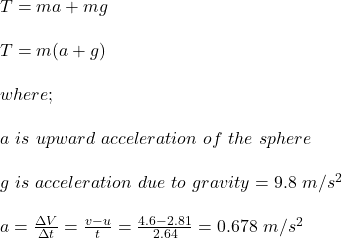## A sphere of mass of 1.55 kg is accelerated upwards by a string to which the sphere is attached. Its speed increases from 2.81 m/s to 4.60 m/

Question

A sphere of mass of 1.55 kg is accelerated upwards by a string to which the sphere is attached. Its speed increases from 2.81 m/s to 4.60 m/s in a time of 2.64 s. Calculate the tension in the string, assuming that the tension remains constant during that time.

in progress 0
2 months 2021-07-23T04:38:43+00:00 1 Answers 4 views 0

The tension in the string is 16.24 N

Explanation:

Given;

mass of the sphere, m = 1.55 kg

initial velocity of the sphere, u = 2.81 m/s

final velocity of the sphere, v = 4.60 m/s

duration of change in the velocity, Δt = 2.64 s

The tension of the string is calculated as follows;T = 1.55(0.678 + 9.8)

T = 1.55(10.478)

T = 16.24 N

Therefore, the tension in the string is 16.24 N